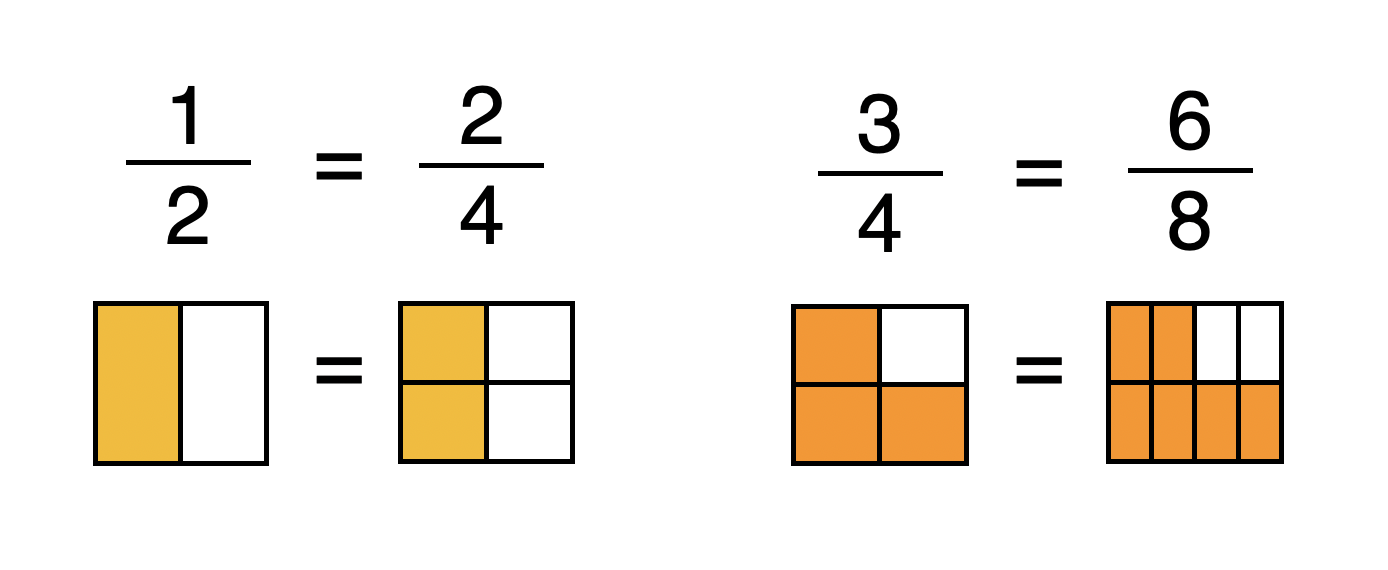# Equivalent Fractions

## What are equivalent fractions?

Equivalent fractions are fractions that are equal in size, even though they have different numerators and denominators.## Fractions equivalent to 1/2:

If the denominator is exactly double the numerator, then the fraction is equivalent to one half.

## Fractions equivalent to 1/3:

If the denominator is exactly three times the numerator, then the fraction is equivalent to one third.

## Fractions equivalent to 1/4:

If the denominator is exactly four times the numerator, then the fraction is equivalent to one quarter.

## How to find equivalent fractions

To find an equivalent fraction of a given fraction, you need to multiply or divide the numerator and denominator by the same number.

## How to find missing numbers in equivalent fractions

1. In this missing number question, we have a missing numerator.

2. Look at the denominators. What would you multiply the first denominator by to make it equal the second denominator?

In this case, 8 x 2 = 16.

3. To find the missing number, we now need to multiply the numerator of the first number by 2.

In this case, 2 x 2 = 4.

The missing numerator is 4.

1. In this missing number question, we have a missing denominator.

2. Look at the numerators. What would you divide the first numerator by to make it equal the second numerator?

In this case, 12 ÷ 4 = 3.

3. To find the missing number, we now need to divide the denominator of the first number by 4.

In this case, 20 ÷ 4 = 5.

The missing denominator is 5.

## Equivalent fractions KS2

In the National Curriculum, equivalent fractions are first introduced in Year 3. Here are the objectives for KS2, taken from the Number - Fractions strand:

## Equivalent fractions Year 3

• recognise and show, using diagrams, equivalent fractions with small denominators

If you are looking for ready-to-teach lessons that cover this objective, you might be interested in our Finding Fractions scheme, or our Fractions in Action scheme for Year 3.

## Equivalent fractions Year 4

• recognise and show, using diagrams, families of common equivalent fractions

If you are looking for ready-to-teach lessons that cover this objective, you might be interested in our Fractions and Time scheme, or our Proportion Problems scheme for Year 4.

## Equivalent fractions Year 5

• identify, name and write equivalent fractions of a given fraction, represented visually, including tenths and hundredths

If you are looking for ready-to-teach lessons that cover this objective, you might be interested in our Fractions and Proportion scheme, or our Decimals and Fractions scheme for Year 5.

## Equivalent fractions Year 6

• add and subtract fractions with different denominators and mixed numbers, using the concept of equivalent fractions

If you are looking for ready-to-teach lessons that cover this objective, you might be interested in our Calculating Fractions and Decimals scheme for Year 6.